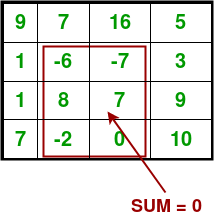# Largest rectangular sub-matrix whose sum is 0

• Difficulty Level : Hard
• Last Updated : 09 Aug, 2022

Given a 2D matrix, find the largest rectangular sub-matrix whose sum is 0. for example consider the following N x M input matrixExamples:

```Input :  1,  2,  3
-3, -2, -1
1,  7,  5

Output : 1,  2,  3
-3, -2, -1

Input :  9,  7, 16,  5
1, -6, -7,  3
1,  8,  7,  9
7, -2,  0, 10

Output :-6, -7
8,  7
-2,  0    ```

The naive solution for this problem is to check every possible rectangle in given 2D array. This solution requires 4 nested loops and time complexity of this solution would be O(n^4).

The solution is based on Maximum sum rectangle in a 2D matrix. The idea is to reduce the problem to 1 D array. We can use Hashing to find maximum length of sub-array in 1-D array in O(n) time. We fix the left and right columns one by one and find the largest sub-array with 0 sum contiguous rows for every left and right column pair. We basically find top and bottom row numbers (which have sum is zero) for every fixed left and right column pair.

To find the top and bottom row numbers, calculate sum of elements in every row from left to right and store these sums in an array say temp[]. So temp[i] indicates sum of elements from left to right in row i. If we find largest subarray with 0 sum on temp, and no. of elements is greater than previous no. of elements then update the values of final row_up, final row_down, final col_left, final col_right.

Implementation:

## CPP

 `// A C++ program to find Largest rectangular``// sub-matrix whose sum is 0``#include ``using` `namespace` `std;` `const` `int` `MAX = 100;` `// This function basically finds largest 0``// sum subarray in temp[0..n-1]. If 0 sum``// does't exist, then it returns false. Else``// it returns true and sets starting and``// ending indexes as starti and endj.``bool` `sumZero(``int` `temp[], ``int``* starti,``            ``int``* endj, ``int` `n)``{``    ``// Map to store the previous sums``    ``map<``int``, ``int``> presum;``    ``int` `sum = 0; ``// Initialize sum of elements` `    ``// Initialize length of sub-array with sum 0``    ``int` `max_length = 0;` `    ``// Traverse through the given array``    ``for` `(``int` `i = 0; i < n; i++)``    ``{``        ``// Add current element to sum``        ``sum += temp[i];` `        ``if` `(temp[i] == 0 && max_length == 0)``        ``{``            ``*starti = i;``            ``*endj = i;``            ``max_length = 1;``        ``}``        ``if` `(sum == 0)``        ``{``            ``if` `(max_length < i + 1)``            ``{``                ``*starti = 0;``                ``*endj = i;``            ``}``            ``max_length = i + 1;``        ``}` `        ``// Look for this sum in Hash table``        ``if` `(presum.find(sum) != presum.end())``        ``{``            ``// store previous max_length so``            ``// that we can check max_length``            ``// is updated or not``            ``int` `old = max_length;` `            ``// If this sum is seen before,``            ``// then update max_len``            ``max_length = max(max_length, i - presum[sum]);` `            ``if` `(old < max_length)``            ``{``                ``// If max_length is updated then``                ``// enter and update start and end``                ``// point of array``                ``*endj = i;``                ``*starti = presum[sum] + 1;``            ``}``        ``}``        ``else` `            ``// Else insert this sum with``            ``// index in hash table``            ``presum[sum] = i;``    ``}` `    ``// Return true if max_length is non-zero``    ``return` `(max_length != 0);``}` `// The main function that finds Largest rectangle``// sub-matrix in a[][] whose sum is 0.``void` `sumZeroMatrix(``int` `a[][MAX], ``int` `row, ``int` `col)``{``    ``int` `temp[row];` `    ``// Variables to store the final output``    ``int` `fup = 0, fdown = 0, fleft = 0, fright = 0;``    ``int` `sum;``    ``int` `up, down;``    ``int` `maxl = INT_MIN;` `    ``// Set the left column``    ``for` `(``int` `left = 0; left < col; left++)``    ``{``        ``// Initialize all elements of temp as 0``        ``memset``(temp, 0, ``sizeof``(temp));` `        ``// Set the right column for the left column``        ``// set by outer loop``        ``for` `(``int` `right = left; right < col; right++)``        ``{``            ``// Calculate sum between current left``            ``// and right for every row 'i'``            ``for` `(``int` `i = 0; i < row; i++)``                ``temp[i] += a[i][right];` `            ``// Find largest subarray with 0 sum in``            ``// temp[]. The sumZero() function also``            ``// sets values of start and finish. So``            ``// 'sum' is sum of rectangle between (start,``            ``// left) and (finish, right) which is``            ``// boundary columns strictly as left and right.``            ``bool` `sum = sumZero(temp, &up, &down, row);``            ``int` `ele = (down - up + 1) * (right - left + 1);` `            ``// Compare no. of elements with previous``            ``// no. of elements in sub-Matrix.``            ``// If new sub-matrix has more elements``            ``// then update maxl and final boundaries``            ``// like fup, fdown, fleft, fright``            ``if` `(sum && ele > maxl)``            ``{``                ``fup = up;``                ``fdown = down;``                ``fleft = left;``                ``fright = right;``                ``maxl = ele;``            ``}``        ``}``    ``}` `    ``// If there is no change in boundaries``    ``// than check if a is 0``    ``// If it not zero then print``    ``// that no such zero-sum sub-matrix exists``    ``if` `(fup == 0 && fdown == 0 && fleft == 0 &&``            ``fright == 0 && a != 0) {``        ``cout << ``"No zero-sum sub-matrix exists"``;``        ``return``;``    ``}` `    ``// Print final values``    ``for` `(``int` `j = fup; j <= fdown; j++)``    ``{``        ``for` `(``int` `i = fleft; i <= fright; i++)``            ``cout << a[j][i] << ``" "``;``        ``cout << endl;``    ``}``}` `// Driver program to test above functions``int` `main()``{``    ``int` `a[][MAX] = { { 9, 7, 16, 5 }, { 1, -6, -7, 3 },``                      ``{ 1, 8, 7, 9 }, { 7, -2, 0, 10 } };`` ` `    ``int` `row = 4, col = 4;``    ``sumZeroMatrix(a, row, col);``    ``return` `0;``}`

Output

```-6 -7
8 7
-2 0 ```

Time Complexity: O(n3)

Auxiliary Space: O(1)

This article is contributed by Harshit Agrawal. If you like GeeksforGeeks and would like to contribute, you can also write an article using write.geeksforgeeks.org or mail your article to review-team@geeksforgeeks.org. See your article appearing on the GeeksforGeeks main page and help other Geeks.

My Personal Notes arrow_drop_up